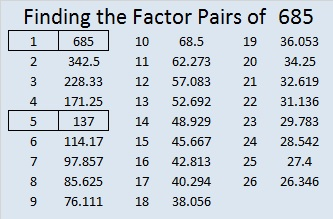# 685 Is the Sum of Two Squares Two Different Ways

Because both of its prime factors are hypotenuses of primitive Pythagorean triples, 685 is the hypotenuse of FOUR Pythagorean triples. Two are primitive; two are not:

• 37-684-685 which was calculated from 19² – 18², 2(19)(18), 19² + 18²
• 156-667-685 which was calculated from 2(26)(3), 26² – 3², 26² + 3²
• 411-548-685 (What factor of 685 is the greatest common factor of those 3 numbers?)
• 440-525-685 (and what is their greatest common factor?)

As you may have notice from those calculations, 685 is the sum of two squares two different ways:

• 19² + 18² = 685
• 26² + 3² = 685

685 is the 19th centered square number because 18 and 19 are consecutive numbers and 19² + 18² = 685. There are 685 small squares of various colors in this graphic.—————————————————————————————————

• 685 is a composite number.
• Prime factorization: 685 = 5 x 137
• The exponents in the prime factorization are 1 and 1. Adding one to each and multiplying we get (1 + 1)(1 + 1) = 2 x 2 = 4. Therefore 685 has exactly 4 factors.
• Factors of 685: 1, 5, 137, 685
• Factor pairs: 685 = 1 x 685 or 5 x 137
• 685 has no square factors that allow its square root to be simplified. √685 ≈ 26.172505.—————————————————————————————————

This site uses Akismet to reduce spam. Learn how your comment data is processed.